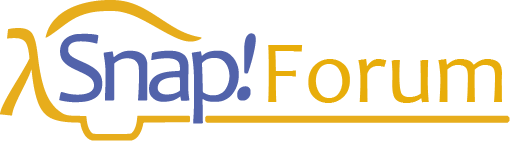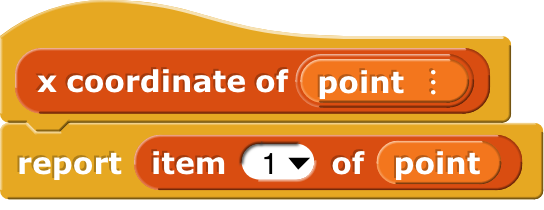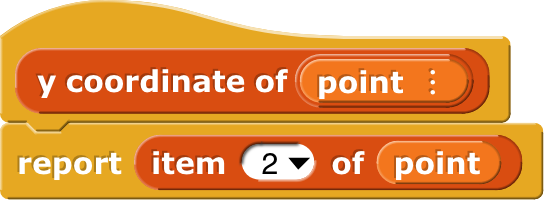# What is the significance of SICP?

I read this, but why should I be interested in SICP as a programmer?
@bh

Oh lord how long a reply do you want?

Let me start here: It's been a decade since I retired from teaching at Berkeley, and I still get a few emails every year from former students saying "I hated 61A [our SICP course] when I took it, but now that I'm actually working in industry I find myself using those ideas every day."

SICP was written to be the first-semester freshman CS course at MIT. Prior to SICP, first CS courses were all about the foibles of some particular programming language, and often named after the language. (Even worse, at some schools, especially two-year community colleges, the second, third, and fourth courses were also named after other programming languages, as if what it means to be a computer scientist is to be able to program in Java and Python and C++ and Fortran.) SICP was the first freshman CS text about ideas, mostly about different aspects of abstraction.

Here's one way to think about it: SICP is the Anti-Scratch. Scratch kids write these awe-inspiring, mile-long scripts full of primitive blocks because, especially in the early days, that's all the language let them do. Such scripts must be a bear to debug, and are impossible to read, no matter how many comments they include. (Scratch has always allowed multiple threads and event-driven code as a form of abstraction, though.) In SICP you learn early on why it's useful to writeeven though a call to one of these blocks takes the same space in the overall program as the one-line ITEM call it replaces.

Of course there are many more elaborate examples of abstraction, but the main lesson is that a human being writing a program is much harder than a computer running the program, so the goal of programming methods is to make the programmer's job easier, by making the language (in the human sense, not the technical sense) available to the programmer more richly able to express ideas.

Okay, that's the overview.

The first ("Structure") half of the book has three chapters, on control structures, data structures, and structures to represent a computation that models a system whose state changes over time.

So, control structures. This chapter could have been called "beyond loops." It starts with recursive functions, which can represent iteration (loop-like, in the case of tail recursion) and branched (tree-like) structures. Recursion allows a mathematical function definition such as

fib(n) = 1, if n≤1
fib(n) = fib(n-1) + fib(n-2), if n>1


to be directly executable as code

(define (fib n)
(if (<= n 1)
1
(+ (fib (- n 1)) (fib (- n 2)))))


(It then points out how terribly inefficient that is, not just a constant factor like you guys' favorite trick of writing in JS instead of in Snap!, but taking exponential time instead of linear time if implemented using a loop. But then it points out that by using the technique of memoization [sic] you can have your cake and eat it too, with code that both shows the tree structure of the underlying idea and runs fast.)

Next up is higher order functions and lambda.

(define (sum term a next b)
(if (> a b)
0
(+ (term a)
(sum term (next a) next b))))


So to find the sum of the first 10 squares you'd say

(sum (lambda (x) (* x x))
1
(lambda (x) (+ x 1))
10)


If you want just the first ten odd squares, you'd use (lambda (x) (+ x 2)) for the next input and 19 for b.

The chapter ends with the use of higher order functions on functions, such as

(define (compose f g)
(lambda (x) (f (g x))))


to do some fancy generalizations of fixed point computations.

Chapter 2 starts by introducing lists, and then uses them to implement increasingly powerful data abstractions, starting with the example I gave in Snap! above about creating a point abstract data type. Here's one of my favorite SICP exercises:

Exercise 2.29. A binary mobile consists of two branches, a left branch and a right branch. Each branch is a rod of a certain length, from which hangs either a weight or another binary mobile. We can represent a binary mobile using compound data by constructing it from two branches (for example, using list):

(define (make-mobile left right)
(list left right))


A branch is constructed from a length (which must be a number) together with a structure, which may be either a number (representing a simple weight) or another mobile:

(define (make-branch length structure)
(list length structure))


a. Write the corresponding selectors left-branch and right-branch, which return the branches of a mobile, and branch-length and branch-structure, which return the components of a branch.

b. Using your selectors, define a procedure total-weight that returns the total weight of a mobile.

c. A mobile is said to be balanced if the torque applied by its top-left branch is equal to that applied by its top-right branch (that is, if the length of the left rod multiplied by the weight hanging from that rod is equal to the corresponding product for the right side) and if each of the submobiles hanging off its branches is balanced. Design a predicate that tests whether a binary mobile is balanced.

d. Suppose we change the representation of mobiles so that the constructors are

(define (make-mobile left right)
(cons left right))
(define (make-branch length structure)
(cons length structure))


How much do you need to change your programs to convert to the new representation?

[Cons is Scheme for in front of.]

I love this exercise because students who try it without paying careful attention to the domain and range of the different procedures fail miserably at writing balanced? because they get mobiles and branches mixed up, and yet it's really easy if you do think in terms of the abstract data types.

The chapter continues with an exploration of how to handle multiple representations for an abstraction, such as rectangular form and polar form of complex numbers. The starting point is to attach explicit type tags to data: (list 'rectangular 3 4) vs. (list 'polar 5 53.13). From there they build up to a complete arithmetic system including the full numeric tower (integer, rational, real, complex) and even symbolic algebra.

Okay, I'm going to speed up now because this is already super long. Chapter 3 introduces OOP not as The Only Way To Program but as an abstraction particularly useful in modeling systems that change over time, e.g., studying how traffic jams happen on highways. But then it introduces streams as a purely functional representation for time-varying state.

The last two chapters ("Interpretation") are about interpreters and compilers. The typical (dragon book) compiler course is mostly about parsing, with hardly anything to say about semantics. One big advantage of using Scheme as the programming language for SICP is that a Scheme program is already data, namely a list of lists. The parentheses that surround a procedure call are exactly the parentheses that surround a sublist in a list. So the SICP treatment of compilation can focus on the interesting part, namely, how to ascribe meaning to the text of a program.

Really, out in the world, there are lots of programmers, but they truly are divided into two kinds, the ones who've read SICP and the muggles.

P.S. I forgot to say, SICP is notoriously hard reading, not so much because the ideas are hard as because they don't waste words. The text is very dense. So you might want to start with Simply Scheme, a SICP prequel.

Is there any difference between reading the book and watching the lectures?

Oh, yes. For one thing, the book has exercises, which you should do! The purpose of a lecture is to show the helicopter view of a topic, highlighting the main points. A lecture doesn't leave you ready to actually use the ideas in your own work. You should do both.